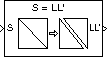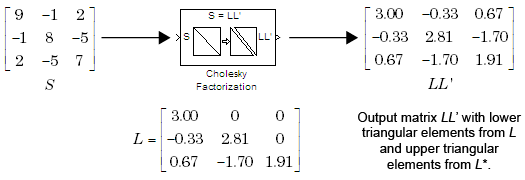# Cholesky Factorization

Factor square Hermitian positive definite matrix into triangular components

## Library

Math Functions / Matrices and Linear Algebra / Matrix Factorizations

`dspfactors`

•## Description

The Cholesky Factorization block uniquely factors the square Hermitian positive definite input matrix S as

`$S=L{L}^{*}$`

where L is a lower triangular square matrix with positive diagonal elements and L* is the Hermitian (complex conjugate) transpose of L. The block outputs a matrix with lower triangle elements from L and upper triangle elements from L*. The output is not in the same form as the output of the MATLAB® `chol` function. In order to convert the output of the Cholesky Factorization block to the MATLAB form, use the following equation:

```R = triu(LL'); ```

In order to extract the L matrix exclusively, pass the output of the Cholesky Factorization block, LL', to the Extract Triangular Matrix block. Setting the Extract parameter of the Extract Triangular Matrix to `Lower` extracts the L matrix. Setting the Extract parameter to `Upper` extracts the L' matrix.

Here, `LL'` is the output of the Cholesky Factorization block. Due to roundoff error, these equations do not produce a result that is exactly the same as the MATLAB result.

Block Output Composed of L and L*### Input Requirements for Valid Output

The block output is valid only when its input has the following characteristics:

• Hermitian — The block does not check whether the input is Hermitian; it uses only the diagonal and upper triangle of the input to compute the output.

• Real-valued diagonal entries — The block disregards any imaginary component of the input's diagonal entries.

• Positive definite — Set the block to notify you when the input is not positive definite as described in Response to Nonpositive Definite Input.

### Response to Nonpositive Definite Input

To generate a valid output, the block algorithm requires a positive definite input (see Input Requirements for Valid Output). Set the Non-positive definite input parameter to determine how the block responds to a nonpositive definite input:

• `Ignore` — Proceed with the computation and do not issue an alert. The output is not a valid factorization. A partial factorization will be present in the upper left corner of the output.

• `Warning` — Display a warning message in the MATLAB Command Window, and continue the simulation. The output is not a valid factorization. A partial factorization will be present in the upper left corner of the output.

• `Error` — Display an error dialog and terminate the simulation.

Note

The Non-positive definite input parameter is a diagnostic parameter. Like all diagnostic parameters on the Configuration Parameters dialog box, it is set to `Ignore` in the code generated for this block by Simulink® Coder™ code generation software.

### Performance Comparisons with Other Blocks

Note that L and L* share the same diagonal in the output matrix. Cholesky factorization requires half the computation of Gaussian elimination (LU decomposition), and is always stable.

## Parameters

Non-positive definite input

Response to nonpositive definite matrix inputs: `Ignore`, `Warning`, or `Error`. See Response to Nonpositive Definite Input.

## References

Golub, G. H., and C. F. Van Loan. Matrix Computations. 3rd ed. Baltimore, MD: Johns Hopkins University Press, 1996.

## Supported Data Types

PortSupported Data Types

S

• Double-precision floating point

• Single-precision floating point

LL`'`

• Double-precision floating point

• Single-precision floating point

 Autocorrelation LPC DSP System Toolbox Cholesky Inverse DSP System Toolbox Cholesky Solver DSP System Toolbox LDL Factorization DSP System Toolbox LU Factorization DSP System Toolbox QR Factorization DSP System Toolbox `chol` MATLAB

See Matrix Factorizations for related information.

## Extended Capabilities

Introduced before R2006a

## SupportGet trial now